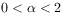Sie sind hier: ICP » R. Hilfer » Publikationen

# 4 Conclusion

[339.1.1] Fractional Bochner-Levy-Riesz diffusion represents an interesting mathematical generalization. [339.1.2] It remains to be seen, however, whether and in which approximation fractional spatial derivatives can arise phenomenologically in mathematical models of an underlying physical reality that obeys spatial locality. [339.1.3] If such an approximation should exist for physical particle transport processes, such that physical particle trajectories can be identified with the mathematical paths of a Levy process, then it seems to be an open problem how to understand the nonlocal dependence of stationary states in these systems on the location of, and particle concentration in distant and remote containers. [339.1.4] It will also be interesting to understand whether and in which sense the experiments are reproducible and how the apparatus can be shielded or isolated from the environment. [339.1.5] Without experimental evidence for the mathematical predictions of fractional potential theory it seems difficult to reconcile nonlocality in space (i.e. the case) with theory and experiment.